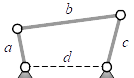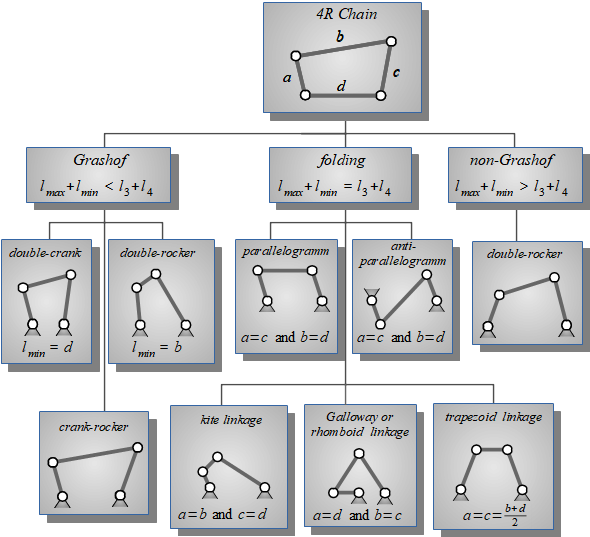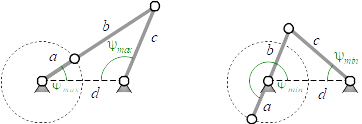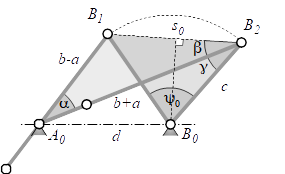## Planar Fourbar Linkages - Fundamental Design Parameters

### Stefan Gössner

Department of Mechanical Engineering, University of Applied Sciences, Dortmund, Germany.

Keywords: R3 chain; crank-rocker; rocker-crank; four-bar; fourbar; planar mechanism; linkage; Grashof; limit angles;

## Abstract

The planar fourbar mechanism is classified according to Grashof's rule. For the practically important crank-rocker mechanism six dependent design parameters are introduced. The relevant constraint equations are derived and discussed.

## Introduction

A fourbar linkage is the most simple mechanism having a mobility of one and revolute joints only. It is composed of four binary links - the bars - connected to each others by four revolute joints. With respect to the latter fact it is also called 4R mechanism.Fig.1: Fourbar Linkage

One of the four links is fixed and called frame. If a link connected to the frame can fully rotate, it is called crank. Otherwise it can only rotate between two angular limits and is called rocker. The floating link opposite to the frame is called coupler and functions as a connecting rod between its neighbour links.

## Design Parameters

The fourbar linkage is uniquely defined by its four link lengths $a, b, c, d$. There are only two restrictions regarding those values.

For successfully assembling the fourbar, the longest link length is required to be less than the sum of the other three link lengths.

$l\_{max} < l\_2 + l\_3 + l\_4$(1)

A fourbar linkage is said to be fully rotatable, if its shortest link is able to perform a complete rotation. Grashof's rule is satisfied then:

$l\_{min} + l\_{max} < l\_3 + l\_4$(2)

Programming hint: Most programming languages offer min and max functions. So by doubling the left sides of inequations (1) and (2) we can code easily

2*max(a,b,c,d) < a + b + c + d   // (1)

2*min(a,b,c,d) + 2*max(a,b,c,d) < a + b + c + d   // (2) Grashof


without having to filter out the remaining link lengths on the right side of (1) and (2).

## Classification

Fourbar linkages can be classified regarding Grashof's rule (2). If there is a less than relationship between the left and right side of equation (2), the fourbar linkage is fully rotatable. If there is a greater than relationship, the linkage is not fully rotatable. In the special case of an equal relationship, the linkage is able to fully rotate. It will take a pose at least twice during one revolution in which all four binary links are collinear. The linkage is said to fold in that configuration (Fig. 2).Fig.2: Classification of Fourbar Linkages

Modler  and Mc.Carthy  have introduced more detailed classification parameters.

## Crank-Rocker

The most important type in practice is undoubtedly the crank-rocker, as it is converting a continuous rotation to a rotational oscillation.Fig.2: Limit positions of the Crank-Rocker

We can identify the two limits on the rocker angle from the positions, where crank and coupler are collinear. Applying the cosine law to each triangle in Fig.2 we get the rocker angle limits

$\\cos\\psi\_{max} = \\frac{c^2 + d^2 - (b+a)^2}{2cd} \\quad and \\quad \\cos\\psi\_{min} = \\frac{c^2 + d^2 - (b-a)^2}{2cd}\\,.$(3)

The associated crank angles can be determined the same way.

$\\cos\\varphi\_{max} = \\frac{-c^2 + d^2 + (b+a)^2}{2(b+a)d} \\quad and \\quad \\cos(\\varphi\_{min}-180^{\\circ}) = \\frac{-c^2 + d^2 + (b-a)^2}{2(b-a)d}$(4)

Much more of interest than the explicite angular limits are the angular ranges during one complete cycle.Fig.3: Angular regions of rocker and crank while moving unidirectional

The rocker's working angular range is named $\\psi\_0$ and corresponds to the crank's angular range $\\varphi\_0$ during tracking motion, when crank and rocker move unidirectional (counterclockwise in Fig.3). On their way back they run in opposite directions.

$\\psi\_0 = \\psi\_{max} - \\psi\_{min} \\space, \\quad \\varphi\_0 = \\varphi\_{min} - \\varphi\_{max} \\quad and \\quad \\alpha = \\varphi\_0 - 180^\\circ$(4)

During a complete cycle of the continuously rotating crank the forward movement of the rocker occupies the crank angle $180^\\circ+\\alpha$, while the backward movement belongs to crank angle $180^\\circ-\\alpha$ (Fig. 3). Using this we can define the time ratio $t\_f/t\_b$ of the rocker moving cycle

$\\frac{t\_f}{t\_b} = \\frac{180^\\circ+\\alpha}{180^\\circ-\\alpha}\\,.$(5)

So if $\\alpha$ is not 0°, the crank needs different times for the rocker's forth and back motion.

## Extended Design Parameter Set

The degree of non-uniformity expressed by the ratio $t\_f/t\_b$ as well as the angular range $\\psi\_0$ of the rocker are very meaningful design parameters from the engineer's point of view.

So adding $\\psi\_0$ and $\\alpha$ to the four link lengths $a, b,c,d$, we now have six design parameters. There must be two constraint equations between them, since only four parameters are sufficient to uniquely define a crank-rocker mechanism.Fig.4: Geometric relations between six design parameters

Applying the cosine theorem twice for $\\Delta A\_0 B\_1 B\_2$ and $\\Delta B\_0 B\_1 B\_2$ with respect to common side $s\_0$ gives (Fig.4)

$(b+a)^2 + (b-a)^2 - 2(b+a)(b-a)\\cos\\alpha = c^2 + c^2 - 2c^2\\cos\\psi\_0$

Simplifying leads to

$a^2 + b^2 - (b^2 -a^2)\\cos\\alpha = c^2(1-\\cos\\psi\_0)$

Using half angle identity $\\cos x = \\cos^2\\frac{x}{2} - \\sin^2\\frac{x}{2}$ finally results in a first constraint equation between the five parameters $a,b,c,\\alpha,\\psi\_0$.

$a^2\\cos^2\\frac{\\alpha}{2} + b^2\\sin^2\\frac{\\alpha}{2} = c^2\\sin^2\\frac{\\psi\_0}{2}$(6)

The half rectangular triangle $\\Delta B\_0 B\_1 B\_2$ in Fig.4 shows relations between the angles $\\psi\_0, \\beta$ and $\\gamma$

$sin(\\beta+\\gamma) = \\cos\\frac{\\psi\_0}{2}\\quad and \\quad cos(\\beta+\\gamma) = \\sin\\frac{\\psi\_0}{2}\\,,$

which can be resolved for $\\cos\\gamma$ using angle addition identities

$cos\\gamma = \\sin\\frac{\\psi\_0}{2}\\cos\\beta + \\cos\\frac{\\psi\_0}{2}\\sin\\beta\\,.$(7)

From triangle $\\Delta A\_0 B\_1 B\_2$ we can extract the trigonometric relations

$s\_0\\sin\\beta = (b-a)\\sin\\alpha\\quad and\\quad (b-a)\\cos\\alpha + s\_0\\cos\\beta = b+a\\quad with\\quad s\_0 = 2 c\\sin\\frac{\\psi\_0}{2}\\,,$

which we can use to eliminate the dependency on $\\beta$ in expression (7)

$2 c \\cos\\gamma = b + a - (b-a)\\cos\\alpha + (b-a)\\frac{\\sin\\alpha}{\\tan\\frac{\\psi\_0}{2}}\\,.$(8)

Finally we apply the cosine law to triangle $\\Delta A\_0 B\_0 B\_2$

$(b+a)^2 + c^2 - 2(b+a)c\\cos\\gamma = d^2\\,,$

from where we can eliminate $\\cos\\gamma$ using expression (8), yielding another relation between the six design parameters

$(b^2-a^2)\\sin(\\frac{\\psi\_0}{2}-\\alpha) = (d^2-c^2)\\sin\\frac{\\psi\_0}{2}\\,.$(9)

When eliminating $c$ from equation (9) with help of equation (6), we get another relation for the five parameters $a,b,d,\\alpha,\\psi\_0$ with a nice similar shape to (6).

$a^2\\cos^2(\\frac{\\psi\_0}{2}-\\frac{\\alpha}{2}) + b^2\\sin^2(\\frac{\\psi\_0}{2}-\\frac{\\alpha}{2}) = d^2\\sin^2\\frac{\\psi\_0}{2}$(10)

With the design of a crank-rocker mechanism we can choose values for four out of six design parameters $a, b, c, d, \\alpha, \\psi\_0$. The missing two can be determined by two constraint equations from (6), (9) or (10).

Note: Please observe Grashof's rule ($a$ is shortest length) as well as the three special cases below !

## Case $\\alpha = \\psi\_0$

Here equations (9) and (10) reduce to the constraints

$a^2 - b^2 = d^2 - c^2\\quad and\\quad a = d\\sin\\frac{\\psi\_0}{2}$(11)

In addition to $\\psi\_0$ and $\\alpha=\\psi\_0$ any combination of two link lengths can be chosen, except $[a,d]$, since the missing length's $b$ and $c$ couldn't be determined then.

## Case $\\alpha = \\frac{\\psi\_0}{2}$

Here equations (9) and (6) reduce to the constraints

$c = d\\quad and\\quad a^2 + b^2\\tan^2\\frac{\\alpha}{2} = 4c^2\\sin\\frac{\\alpha}{2}$(12)

In addition to $\\psi\_0$ and $\\alpha=\\frac{\\psi\_0}{2}$ any combination of two link lengths can be chosen, except $[c,d]$, as the missing length's $a$ and $b$ couldn't be determined then.

## Case $\\alpha = 0$

Here equations (9) and (6) reduce to the constraints

$b^2 - a^2 = d^2 - c^2\\quad and\\quad a = c\\sin\\frac{\\psi\_0}{2}$(13)

In addition to $\\psi\_0$ and $\\alpha=0$ any combination of two link lengths can be chosen, except $[a,c]$, as the missing length's $b$ and $d$ couldn't be determined then.

The resulting fourbar is called centric fourbar and has interesting and mostly advantageous properties.

## Conclusion

From six meaningful design parameters for crank-rocker mechanisms only four are independent. The relevant constraint equations were derived. With those equations the two missing parameters can be calculated in general. Three special cases are discussed.

## References

 Gössner S., Mechanismentechnik – Vektorielle Analyse ebener Mechanismen, Logos, Berlin, 2016

 McCarthy J.M. et al., Geometric Design of Linkages, Springer, New York, 2010

 Modler K.H., Luck K., Getriebetechnik Analyse, Synthese, Optimierung, Springer, Berlin/Heidelberg, 1995

 Uicker, J.J. et. al., Theory of Machines and Mechanisms, Oxford Press 2011

 VDI-Richtline 2145, Ebene viergliedrige Getriebe mit Dreh- und Schub­gelen­ken, Beuth Berlin, 1980.